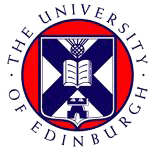留学小程序爱丁堡大学

Artificial IntelligenceMSc

REQUIREMRNT

A UK 2:1 honours degree, or its international equivalent, in informatics, artificial intelligence, cognitive science, computer science, electrical engineering, linguistics, mathematics, philosophy, physics or psychology, plus experience in computer programming. During your degree you must have completed the equivalent to 60 credits of mathematics that have typically covered the following subjects/topics: Calculus (differentiation and integration), Linear Algebra (vectors and multi-dimensional matrices), Discrete Mathematics and Mathematical reasoning (eg. Induction and reasoning, Graph theoretic models, proofs), and Probability (concepts in discrete and continuous probabilities, Markov Chains etc. )

English language requirements

IELTS Academic: total 6.5 with at least 6.0 in each component； TOEFL-iBT: total 92 with at least 20 in each section